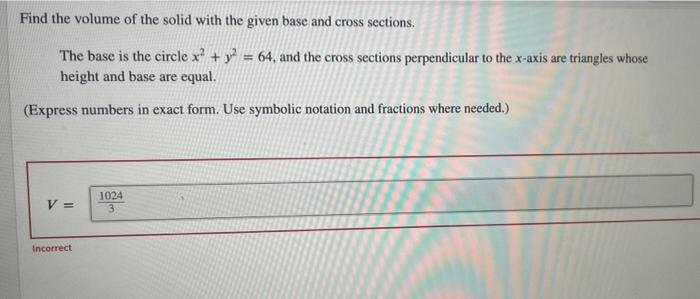# Question Solved1 AnswerFind the volume of the solid with the given base and cross sections. The base is the circle x2 + y2 = 64, and the cross sections perpendicular to the x-axis are triangles whose height and base are equal. (Express numbers in exact form. Use symbolic notation and fractions where needed.) V = 1024 3 IncorrectTranscribed Image Text: Find the volume of the solid with the given base and cross sections. The base is the circle x2 + y2 = 64, and the cross sections perpendicular to the x-axis are triangles whose height and base are equal. (Express numbers in exact form. Use symbolic notation and fractions where needed.) V = 1024 3 Incorrect
More
Transcribed Image Text: Find the volume of the solid with the given base and cross sections. The base is the circle x2 + y2 = 64, and the cross sections perpendicular to the x-axis are triangles whose height and base are equal. (Express numbers in exact form. Use symbolic notation and fractions where needed.) V = 1024 3 Incorrect